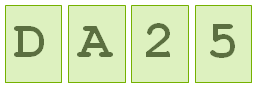### Bayesian Cognitive Bias

I chanced on this excellent puzzle on the net that tends to reveal a cognitive bias in our heads against Bayesian reasoning. The puzzle statement is quite simple

You are given four cards. Each card has a letter on one side and number on the other side. You are told the statement "If there is a D on one side, there is a 5 on the other side". Which two cards would you flip over to validate the statement?The original article is here, think hard before you click through for an answer :)

1.### The Best Books to Learn Probability

If you are looking to buy some books in probability here are some of the best books to learn the art of Probability The Probability Tutoring Book: An Intuitive Course for Engineers and Scientists (and Everyone Else!) A good book for graduate level classes: has some practice problems in them which is a good thing. But that doesn't make this book any less of buy for the beginner. An Introduction to Probability Theory and Its Applications, Vol. 1, 3rd Edition This is a two volume book and the first volume is what will likely interest a beginner because it covers discrete probability. The book tends to treat probability as a theory on its own Discovering Statistics Using R This is a good book if you are new to statistics & probability while simultaneously getting started with a programming language. The book supports R and is written in a casual humorous way making it an easy read. Great for beginners. Some of the data on the companion website could be missing. Fifty Cha

### Fun with Uniform Random Numbers

Q: You have two uniformly random numbers x and y (meaning they can take any value between 0 and 1 with equal probability). What distribution does the sum of these two random numbers follow? What is the probability that their product is less than 0.5. The Probability Tutoring Book: An Intuitive Course for Engineers and Scientists A: Let z = x + y be the random variable whose distribution we want. Clearly z runs from 0 to 2. Let 'f' denote the uniform random distribution between [0,1]. An important point to understand is that f has a fixed value of 1 when x runs from 0 to 1 and its 0 otherwise. So the probability density for z, call it P(z) at any point is the product of f(y) and f(z-y), where y runs from 0 to 1. However in that range f(y) is equal to 1. So the above equation becomes From here on, it gets a bit tricky. Notice that the integral is a function of z. Let us take a look at how else we can simply the above integral. It is easy to see that f(z-y) = 1 when (

### The Best Books for Linear Algebra

The following are some good books to own in the area of Linear Algebra. Linear Algebra (2nd Edition) This is the gold standard for linear algebra at an undergraduate level. This book has been around for quite sometime a great book to own. Linear Algebra: A Modern Introduction Good book if you want to learn more on the subject of linear algebra however typos in the text could be a problem. Linear Algebra (Dover Books on Mathematics) An excellent book to own if you are looking to get into, or want to understand linear algebra. Please keep in mind that you need to have some basic mathematical background before you can use this book. Linear Algebra Done Right (Undergraduate Texts in Mathematics) A great book that exposes the method of proof as it used in Linear Algebra. This book is not for the beginner though. You do need some prior knowledge of the basics at least. It would be a good add-on to an existing course you are doing in Linear Algebra. Linear Algebra, 4th Ed